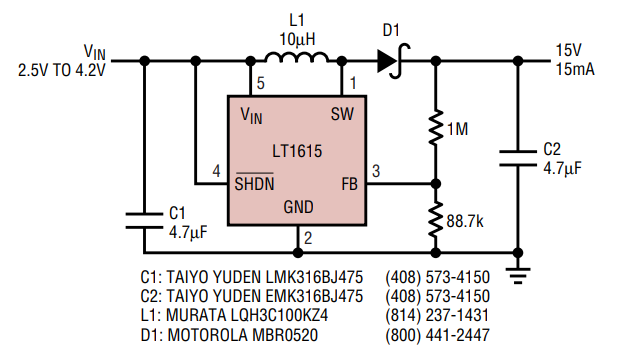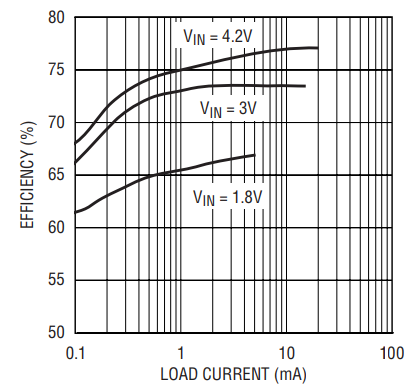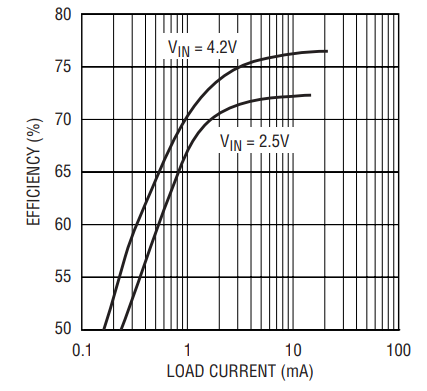# Micropower 5-Lead SOT-23 Switching Regulators Extend Battery Life in Space-Sensitive Applications

Introduction

The LT1615 and LT1617 are designed for portable electronics that need a power solution with a minimum footprint and long battery life. These devices can be used as step-up or boost converters, single-ended primary inductance converters (SEPIC) or positive-to-negative converters. With an input voltage range of 1.2V to 15V, these devices are ideal for a wide range of applications and work with a variety of input sources. An internal 36V switch allows the two devices to easily generate output voltages of up to ±34V without the use of costly transformers. The LT1615 is designed to regulate positive output voltages, whereas the LT1617 is designed to directly regulate negative output voltages without the need for level-shifting circuitry. Both parts use a current-limited, fixed off-time control scheme, which helps achieve high efficiency operation over a wide range of load currents. With a no-load quiescent current of only 20µA (with the output in regulation) and a shutdown quiescent current of 0.5µA, these devices squeeze the most life out of any battery application. Both devices use tiny, low profile inductors and capacitors to minimize the overall system footprint and cost.

The LT1615 and LT1617 are pin compatible with two other members of the PowerSOT family, the LT1613 and LT1611, respectively. This allows the same board layout to be used to evaluate the performance of multiple devices. The LT1613 and LT1611 are both current mode, constant frequency devices, capable of producing larger output currents than the LT1615 and LT1617.1

LT1615 2-Cell to 3.3V Boost Converter

A popular supply for many portable electronic devices, a 2-cell alkaline to 3.3V converter with the LT1615 can deliver 60mA of load current. The circuit is shown in Figure 1 and the system efficiency appears in Figure 2. The efficiency peaks at 84% with a fresh 2-cell battery and averages 78% over the entire 1.5V to 3V input voltage range. Switching waveforms with an input voltage of 2.8V and a 60mA load appear in Figure 3. This photo illustrates the Burst Mode operation of the LT1615, as the system delivers energy to the output in short bursts about every 15µs. The device is in standby (drawing only 20µA of quiescent current) for about 12µs of each 15µs burst cycle, which greatly increases the overall converter efficiency.Figure 1. 2-cell to 3.3V boost converter.Figure 2. 3.3V boost converter efficiency reaches 84%.Figure 3. Switching waveforms of 3.3V boost converter with 60mA load.

LT1615 1-Cell Li-Ion to 15V Boost Converter

The internal 36V switch of the LT1615 makes it an attractive choice for applications that need a high output voltage at a relatively low current. Figure 4’s circuit is a typical system that provides 15V at 15mA from a single-cell Li-Ion battery. Efficiency, shown in Figure 5, reaches 82% from a fully charged battery.Figure 4. Li-Ion to 15V boost converter.Figure 5. Efficiency of Figure 4’s boost converter.

LT1615 33V Boost Converter

The converter in Figure 6 displays the exceptional input and output voltage range of the LT1615. A 33V output is easily generated from a wide ranging input voltage using a simple boost topology. A small, 1µF ceramic capacitor is all that is needed at the output, making the total footprint much smaller than other systems that need much larger tantalum capacitors. The efficiencies for inputs of 3.3V, 5V and 12V are shown in Figure 7. With a 12V input, this converter can deliver up to 1.32W (40mA at 33V) of power at an efficiency of 85%, all from a tiny SOT-23 package.Figure 6. 33V boost converter.Figure 7. 33V boost converter efficiency.

LT1615 1-Cell Li-Ion to 3.3V SEPIC

Lithium-ion (Li-Ion) is the battery of choice for systems needing the most energy with the lightest weight, but with a cell voltage ranging from 4.2V down to 2.5V, a simple boost topology cannot be used to provide a 3.3V output. Figure 8’s circuit is a SEPIC converter that can easily do the job, providing 100mA of load current. The circuit shown uses two separate inductors, but a single, dual-winding inductor (a 1:1 transformer) can be substituted. Figure 9 shows the switching waveforms for this SEPIC converter with an input voltage of 2.7V and a 50mA load. Notice that this circuit uses the same basic Burst Mode operation as the boost converter, but the inductor current is about one half that of the 3.3V boost circuit whose waveforms are shown in Figure 3. For the SEPIC, the switch current is split equally between the two inductors, with both inductors providing current to the load when the switch is turned off. Typical efficiency for this converter is 70%.Figure 8. Li-Ion to 3.3V SEPIC converter.Figure 9. Switching waveforms of the 3.3V SEPIC converter with 50mA load.

LT1615 ±20V Dual-Output Converter

Figure 10 shows a single-inductor, dual-output converter ideal for use in applications needing both a positive and a negative voltage. The positive output is generated using a traditional boost converter, whereas the negative output is generated using an inverting charge pump. Regulation is achieved by sensing the positive output, but by using identical output capacitors and rectifying diodes, the negative output is also very well regulated. For a 2× difference in output currents, the positive and negative output voltages differ less than 3%; for a 10× difference, they differ less than 5%. This converter provides 8mA total output current from a 1.5V input (two fully discharged alkaline batteries) and 12mA total output current from a 2.5V input (a fully discharged single-cell Li-Ion battery). Increasing the value of L1 to 22µH increases the available output current by about 15%. If even larger load currents are needed, the same converter can be implemented using the LT1613 in place of the LT1615. If the accuracy of the negative output is more critical than the accuracy of the positive output, try the same topology using the LT1617 to regulate the negative output. Efficiency for this dual-output converter is quite good, reaching 77% with a single Li-Ion battery. See Figure 11 for efficiency curves at several different input voltages.Figure 10. ±20V dual-output converter.Figure 11. Efficiency of Figure 10’s circuit.

LT1615 ±20V Dual-Output Converter with Load Disconnect

One drawback to the circuit shown in Figure 10 is that during shutdown, the positive output is one diode drop below the input voltage. This is an undesirable condition for many systems, and can be easily corrected with the circuit in Figure 12. This is a dual-output converter where both outputs are developed using charge pumps, so that both are disconnected from the input when the LT1615 is turned off. An additional benefit is that cross regulation is improved because both outputs are generated in the same manner. For a 5× difference in output currents, the positive and negative output voltages differ less than 1%; for a 10× difference, they differ less than 2%. The improvements this circuit provides do come at a cost: slightly lower efficiency. Figure 13 shows that the efficiency curves are about 3% lower for load currents greater than 1mA, but the efficiency still reaches a respectable 74%.Figure 12. ±20V dual-output converter with load disconnect.Figure 13. Efficiency of Figure 12’s circuit.

LT1617 1-Cell Li-Ion to –15V Inverting Converter

Many electronic systems need a negative supply but have only a positive input voltage to work with. A well regulated, positive-to-negative converter can be easily designed using the LT1617. A Li-Ion to –15V inverting converter capable of providing 15mA of load current is detailed in Figure 14. Efficiency for this inverter, shown in Figure 15, peaks at 76%.Figure 14. Li-Ion to –15V inverting converter.Figure 15. Li-Ion to –15V inverting converter efficiency.

LT1617 5V to –33V Inverting Charge Pump

For the previous inverting converter, the maximum voltage seen by the power switch is equal to the sum of the input and output voltages; this, along with the 36V switch rating, limits the output voltage that can be provided using the inverting topology. If higher negative voltages are needed, use an inverting charge pump, in which the maximum voltage seen by the switch is equal to the output voltage. Figure 16 shows a –33V, 20mA inverting charge pump that can provide 20mA of load current. Efficiency reaches 74%, as seen in Figure 17.Figure 16. –33V inverting charge pump.Figure 17. 5V to –33V inverting charge pump efficiency.

Conclusion

The applications presented show the versatility of the LT1615 and LT1617. These devices are capable of producing a wide range of positive and negative outputs from a variety of input sources. Their tiny SOT-23 packages, along with small external components, combine to minimize footprint and cost in space-conscious applications.

1. Pietkiewicz, Steve. “SOT-23 Switching Regulators Deliver Low Noise Outputs in a Small Footprint.” Linear Technology IX:1 (February 1999), pp.11–13, 23.